Case Based Questions (MCQ)

Chapter 12 Class 12 Linear Programming
Serial order wise

## An aeroplane can carry a maximum of 200 passengers.  A profit of Rs. 1000 is made on each executive class  ticket and a profit of Rs. 600 is made on each economy  class ticket. The airline reserves at least 20 seats for  the executive class. However, at least 4 times as many  passengers prefer to travel by economy class, than by  executive class. It is given that the number of executive class tickets is x and that of economy class tickets is y.

This question is inspired from Misc 5 - Chapter 12 Class 12 (Linear Programming)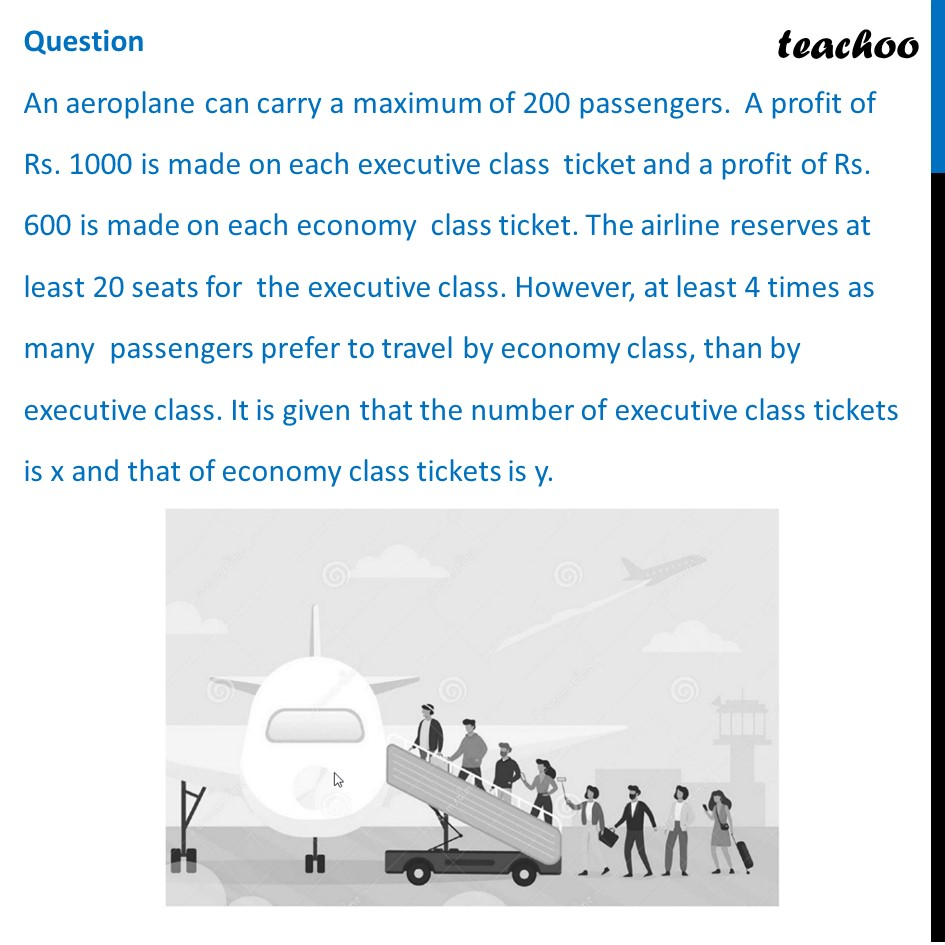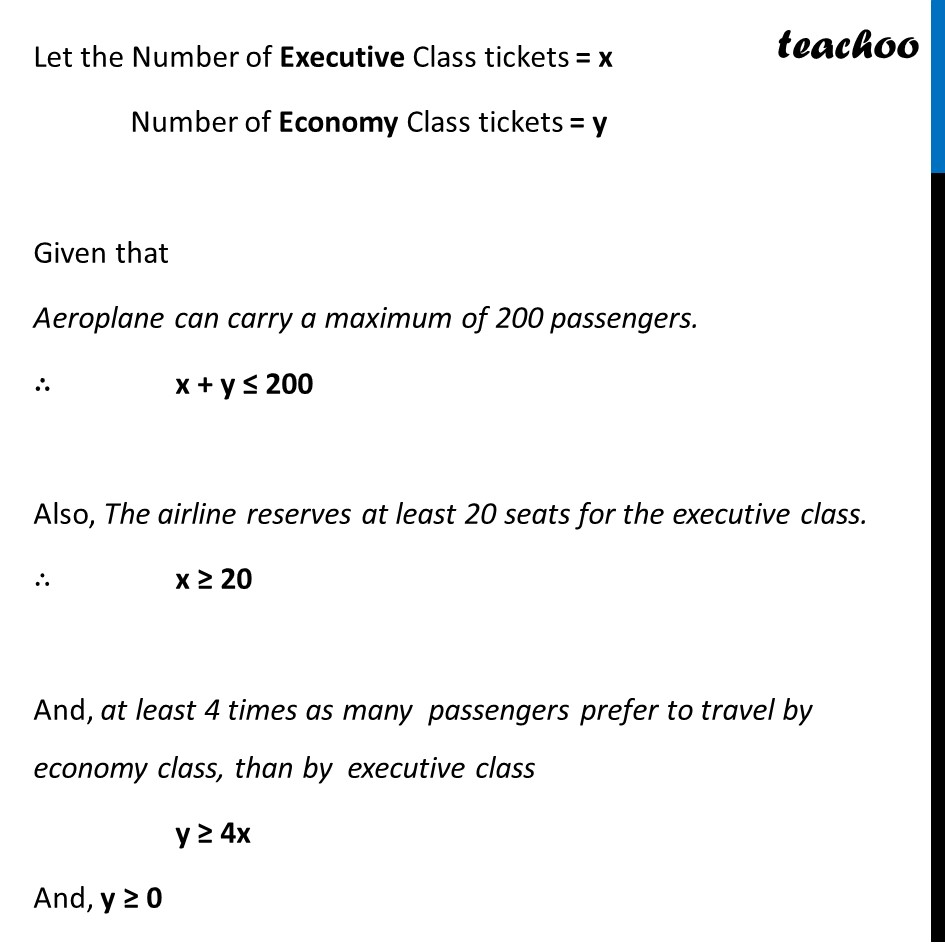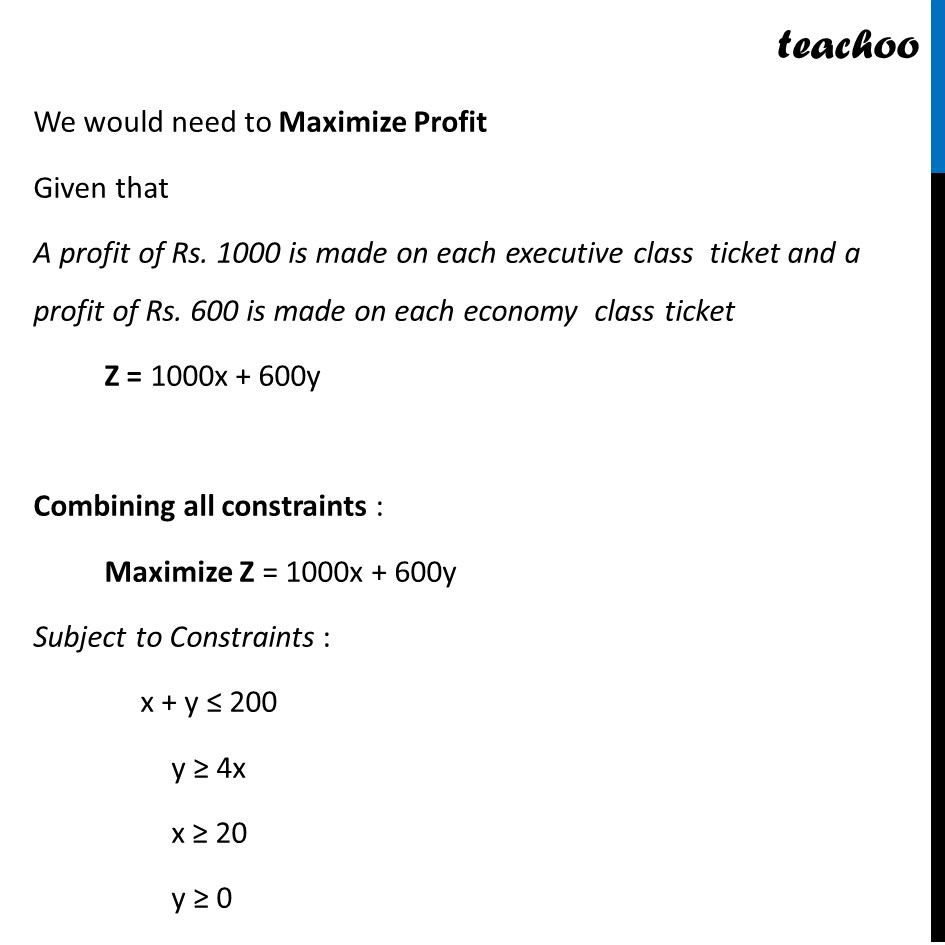## (d) 80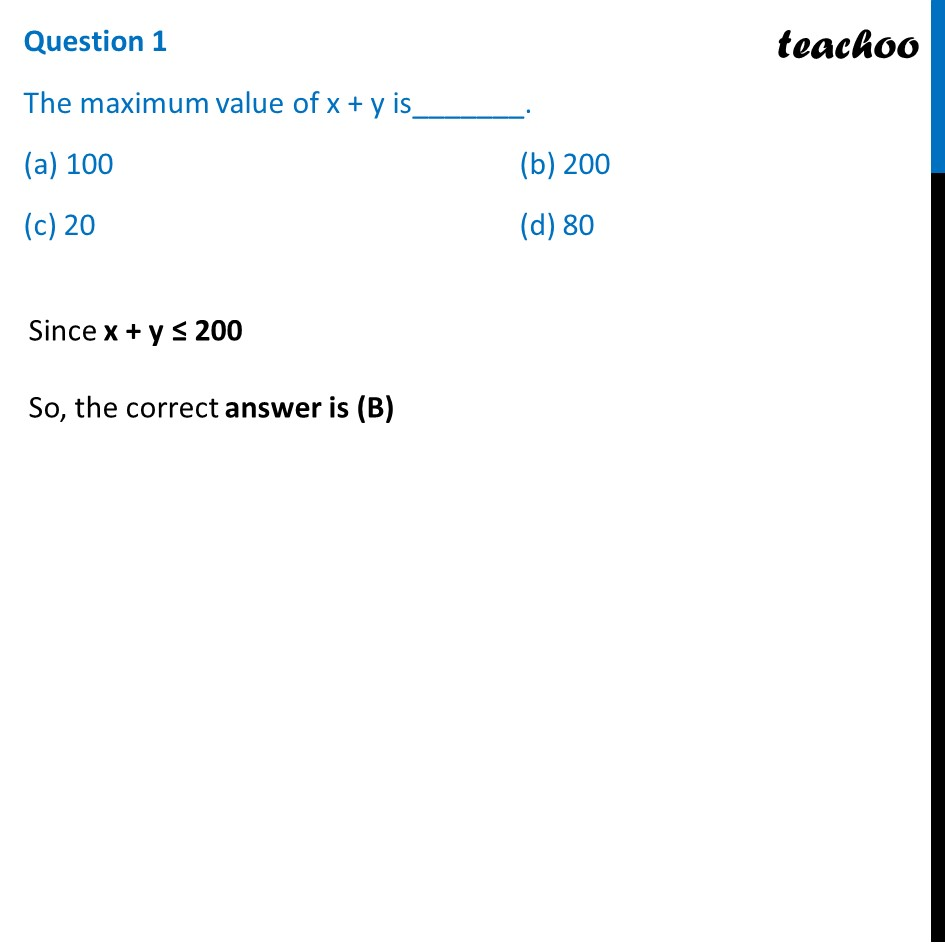## (d) y ≥ 4x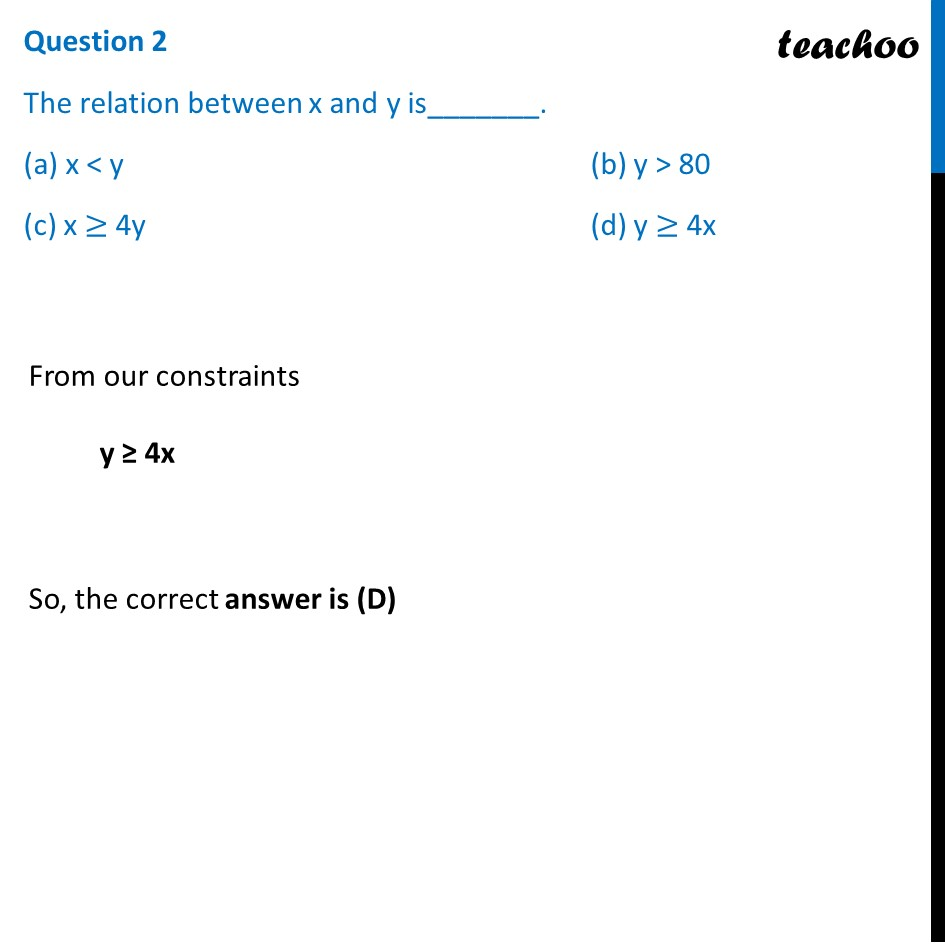## (d) 4x − y ≤ 0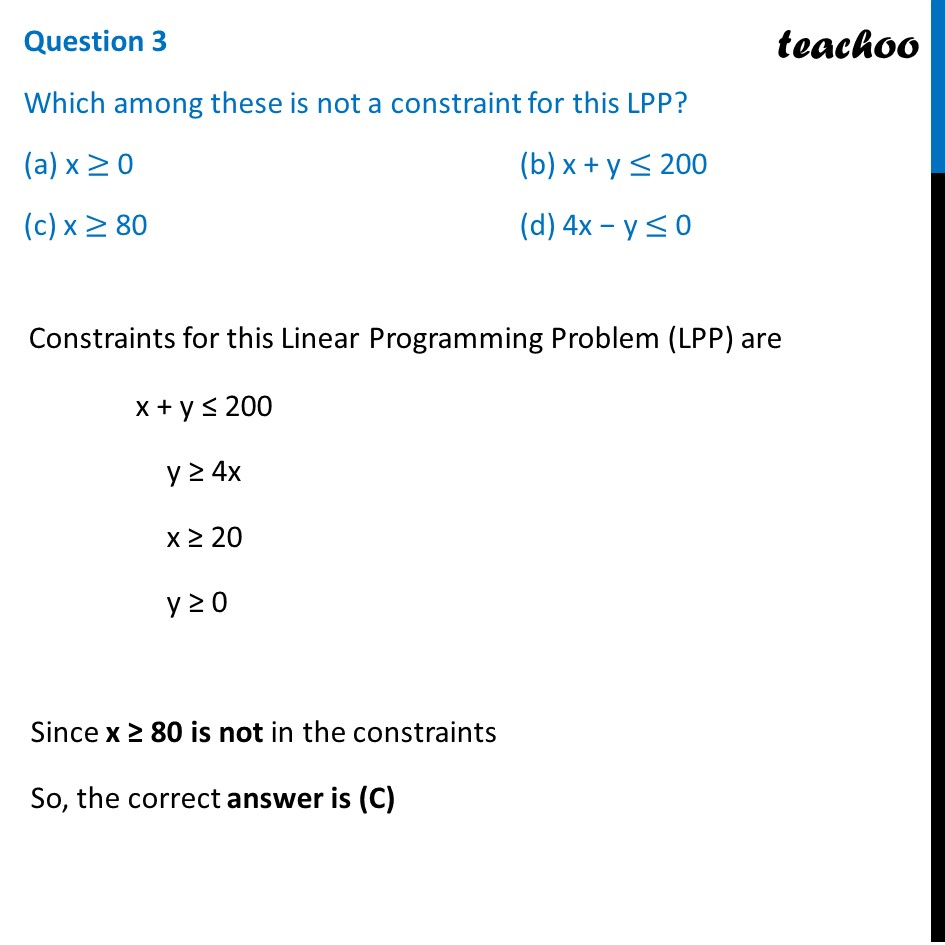## (d) Rs. 136000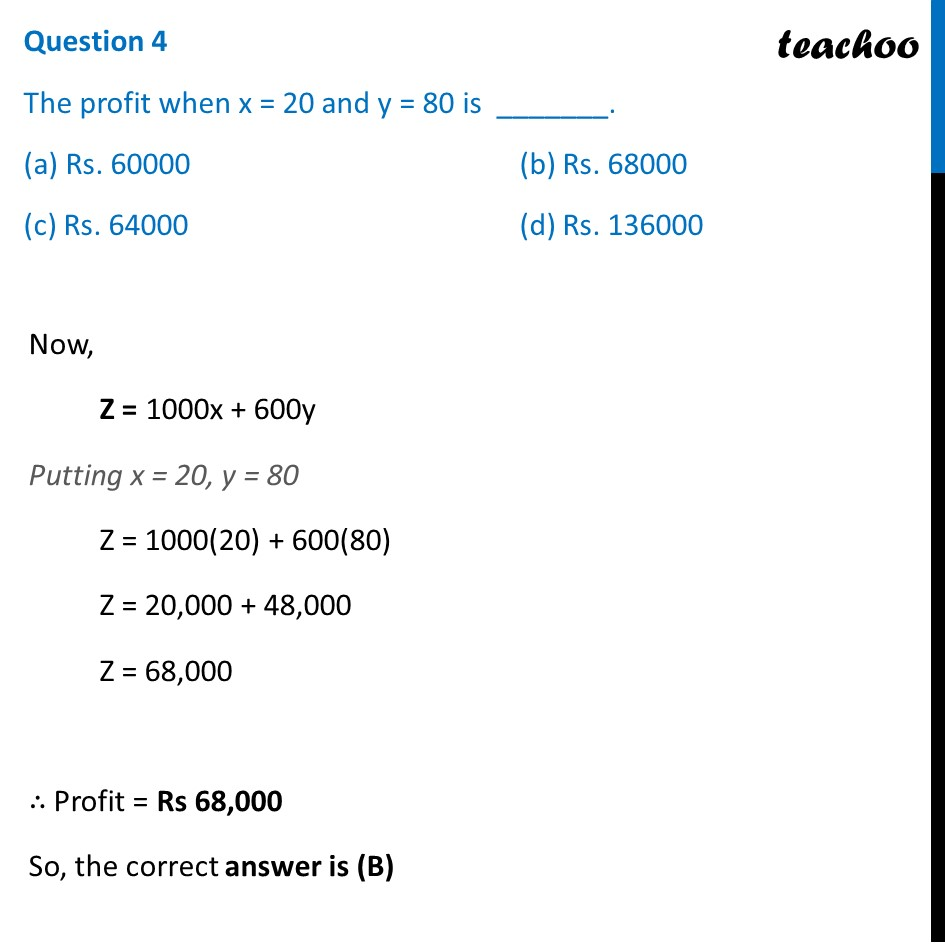## (d) 120000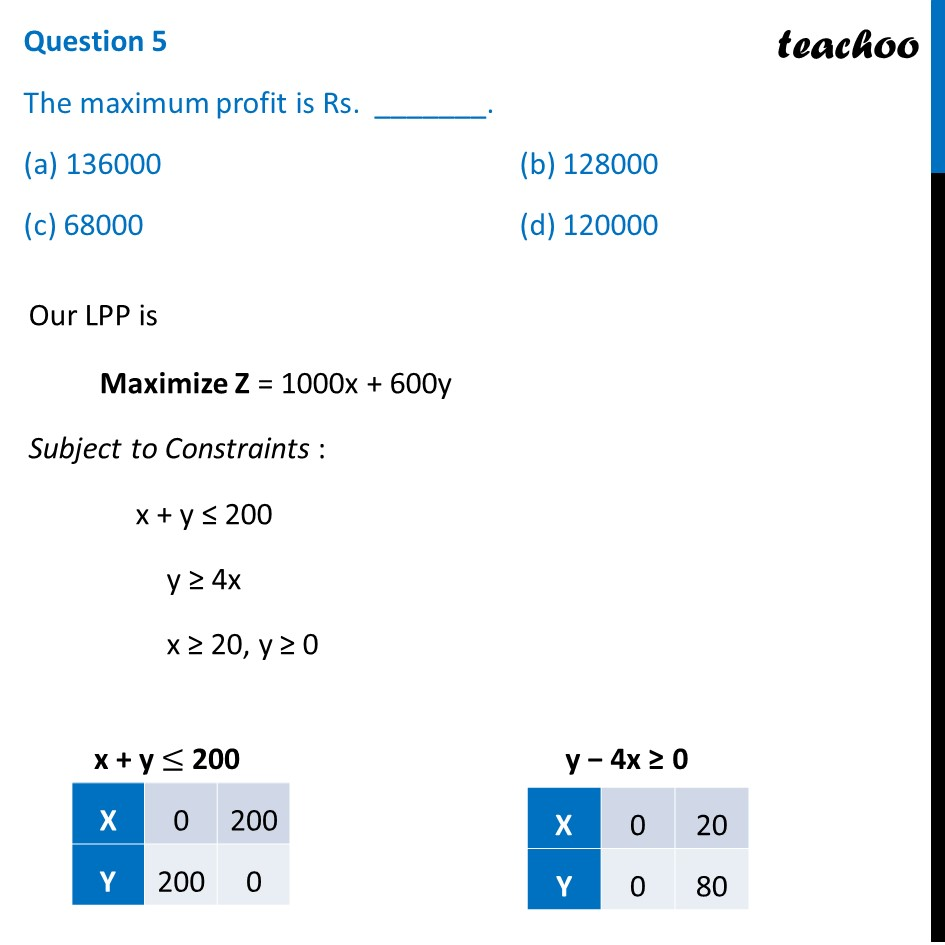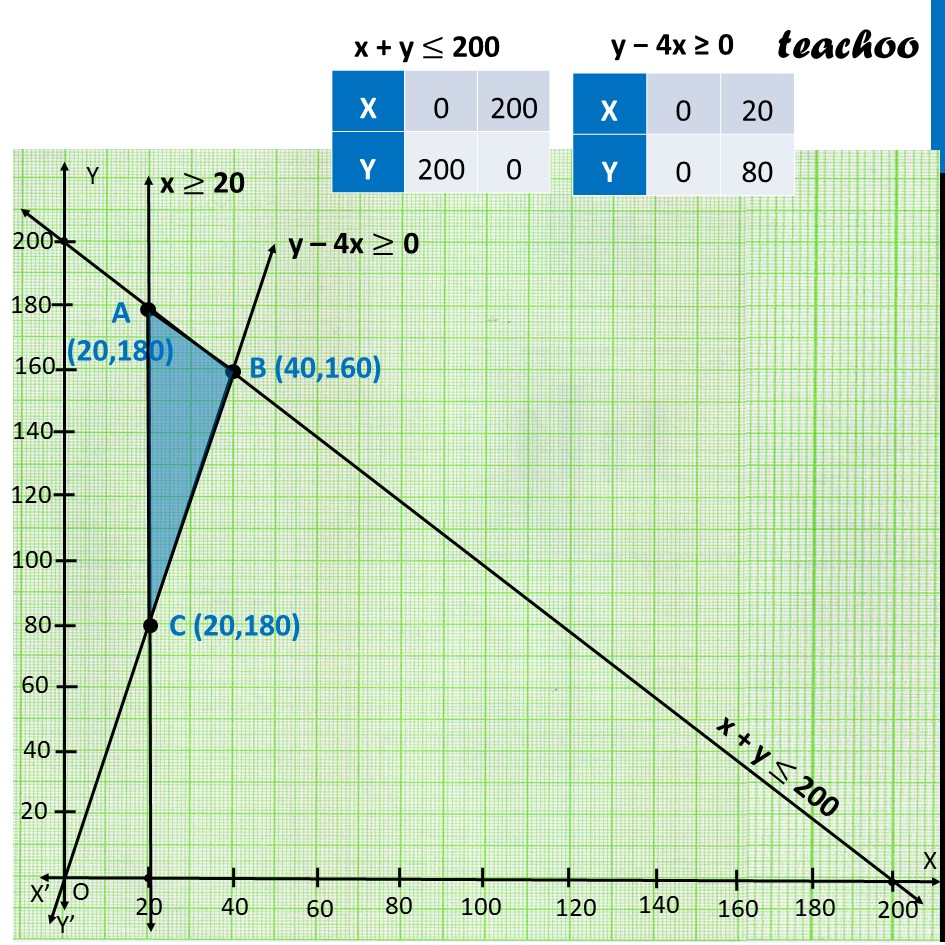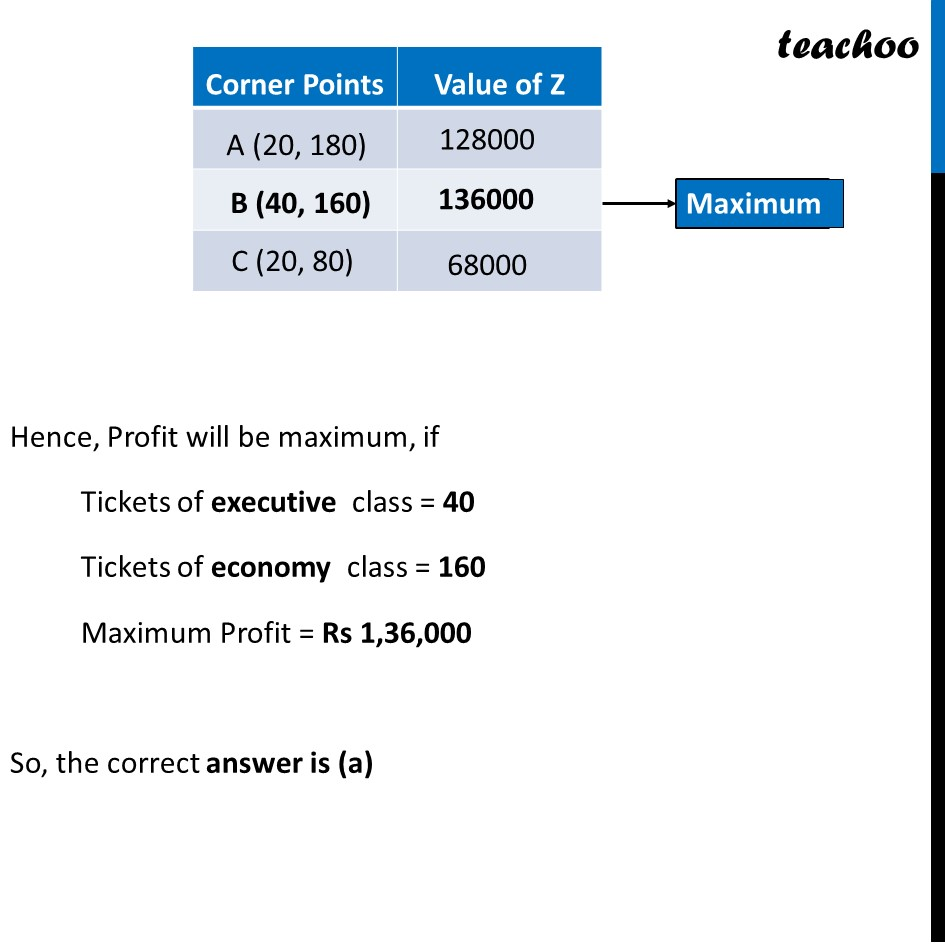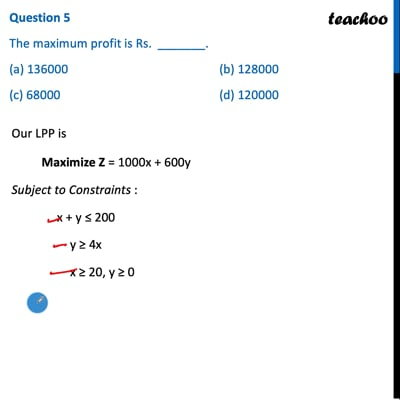This video is only available for Teachoo black users

Maths Crash Course - Live lectures + all videos + Real time Doubt solving!

### Transcript

Question An aeroplane can carry a maximum of 200 passengers. A profit of Rs. 1000 is made on each executive class ticket and a profit of Rs. 600 is made on each economy class ticket. The airline reserves at least 20 seats for the executive class. However, at least 4 times as many passengers prefer to travel by economy class, than by executive class. It is given that the number of executive class tickets is x and that of economy class tickets is y. Let the Number of Executive Class tickets = x Number of Economy Class tickets = y Given that Aeroplane can carry a maximum of 200 passengers. ∴ x + y ≤ 200 Also, The airline reserves at least 20 seats for the executive class. ∴ x ≥ 20 And, at least 4 times as many passengers prefer to travel by economy class, than by executive class y ≥ 4x And, y ≥ 0 We would need to Maximize Profit Given that A profit of Rs. 1000 is made on each executive class ticket and a profit of Rs. 600 is made on each economy class ticket Z = 1000x + 600y Combining all constraints : Maximize Z = 1000x + 600y Subject to Constraints : x + y ≤ 200 y ≥ 4x x ≥ 20 y ≥ 0 Question 1 The maximum value of x + y is_______. (a) 100 (b) 200 (c) 20 (d) 80 Since x + y ≤ 200 So, the correct answer is (B) Question 2 The relation between x and y is_______. (a) x < y (b) y > 80 (c) x ≥ 4y (d) y ≥ 4x From our constraints y ≥ 4x So, the correct answer is (D) Question 3 Which among these is not a constraint for this LPP? (a) x ≥ 0 (b) x + y ≤ 200 (c) x ≥ 80 (d) 4x − y ≤ 0 Constraints for this Linear Programming Problem (LPP) are x + y ≤ 200 y ≥ 4x x ≥ 20 y ≥ 0 Since x ≥ 80 is not in the constraints So, the correct answer is (C) Question 4 The profit when x = 20 and y = 80 is _______. (a) Rs. 60000 (b) Rs. 68000 (c) Rs. 64000 (d) Rs. 136000 Now, Z = 1000x + 600y Putting x = 20, y = 80 Z = 1000(20) + 600(80) Z = 20,000 + 48,000 Z = 68,000 ∴ Profit = Rs 68,000 So, the correct answer is (B) Question 5 The maximum profit is Rs. _______. (a) 136000 (b) 128000 (c) 68000 (d) 120000 Our LPP is Maximize Z = 1000x + 600y Subject to Constraints : x + y ≤ 200 y ≥ 4x x ≥ 20, y ≥ 0 Hence, Profit will be maximum, if Tickets of executive class = 40 Tickets of economy class = 160 Maximum Profit = Rs 1,36,000 So, the correct answer is (a)